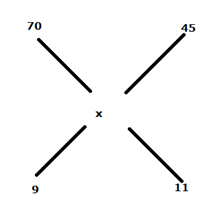# IBPS PO Prelims Quantitative Aptitude Questions 2021 (Day-08)

Dear Aspirants, Our IBPS Guide team is providing new series of Quantitative Aptitude Questions for IBPS PO Prelims 2021 so the aspirants can practice it on a daily basis. These questions are framed by our skilled experts after understanding your needs thoroughly. Aspirants can practice these new series questions daily to familiarize with the exact exam pattern and make your preparation effective.

Start Quiz

Missing number series

Directions (01-05): What value should come in the place of (?) in the following number series?

1) 48, 72, 144, 360, 1080, ?

A.2360

B.3072

C.3544

D.2180

E.3780

2) 1, 4, 20, 120,?, 6720

A.840

B.856

C.720

D.732

E.680

3) 62, 122, 212, 338, 506, ?

A.722

B.738

C.714

D.706

E.762

4) 20, 28, 37, 101, 126, ?

A.325

B.342

C.337

D.320

E.328

5) 23, 33, 63, 113, ?, 273

A.163

B.143

C.183

D.193

E.153

Train

6) A train crosses a man is running in the opposite direction at the speed of 30 kmph in 14.4 seconds and the speed of train is 60 kmph. What is the time taken by train crosses 240 m long platform?

A.24 seconds

B.36 seconds

C.18 seconds

D.14.4 seconds

E.48 seconds

Profit and loss

7) The sum of the cost price of mobile and laptop is Rs.9900. If the shopkeeper sold the mobile at 10% profit and laptop at 8% loss and he found that the loss incurred by selling laptop is equal to the profit earned by selling mobile, then find the cost price of mobile?

A.Rs.4000

B.Rs.4400

C.Rs.4800

D.Rs.5200

E.None of these

Percentage

8) Ram spends 40% of his salary on house rent and the rest of his salary spends on education and shopping. The amount spends on education is 40% more than the amount spends on shopping. If sum of the 20% of the amount spends on house rent and the amount on education is Rs.68800, then find the salary of Ram?

A.Rs.180000

B.Rs.150000

C.Rs.120000

D.Rs.160000

E.None of these

Mixture and allegation

9) The price of A1 quality rice is Rs.70 per kg and the price of A2 quality rice is Rs.45 per kg. If both quality of rice are mixed in the ratio of 9:11, then what is the price per kg of the mixture?

A.Rs.58.75

B.Rs.56.80

C.Rs.54.75

D.Rs.52.90

E.Rs.50.45

Probability

10) A group of 6 teachers is to be selected from 10 male and 8 female teachers in the school. What is the probability of that group has at least 4 female teachers?

A.89/442

B.23/442

C.19/442

D.17/442

E.None of these

### Try IBPS PO Prelims Free Online Mock Test

48 * 1.5 = 72

72 * 2 = 144

144 * 2.5 = 360

360 * 3 = 1080

1080 * 3.5 = 3780

1 * 4 = 4

4 * 5 = 20

20 * 6 = 120

120 * 7 = 840

840 * 8 = 6720

43 – 2 = 62

53 – 3 = 122

63 – 4 = 212

73 – 5 = 338

83 – 6 = 506

93 – 7 = 722

20 + 23 = 28

28 + 32 = 37

37 + 43 = 101

101 + 52 = 126

126 + 63 = 342

23 + 10 = 33

33 + 30 = 63

63 + 50 = 113

113 + 70 = 183

183 + 90 = 273

Length of train = (30 + 60) * 5/18 * 14.4

= 360 m

Required time = (360 + 240)/(60 * 5/18)

= 36 seconds

CP of mobile = x

CP of laptop = 9900 – x

SP of laptop = (9900 – x) * 92/100

SP of mobile = x * 110/100

x * 10/100 = (9900 – x) * 8/100

10x = 79200 – 8x

x = 4400

Amount spends on shopping = 5x

Amount spends on education = 5x * 140/100 = 7x

Salary of Ram = 100/60 * 12x = 20x

Amount spends on house rent = 20x * 40/100 = 8x

8x * 20/100 + 7x = 68800

8x + 35x = 68800 * 5

x = 8000

Ram salary = 8000 * 20 = Rs.160000(70 – x)/(x – 45) = 9/11

9x – 405 = 770 – 11x

20x = 1175

x = 58.75

Required probability = ((8C4 * 10C2) + (8C5 * 10C1) + 8C6)/(18C6)

= ((70 * 45) +(56*10)+ 28)/(17 * 6 * 14 * 13)

= 89/442

For More Days IBPS PO Prelims Practice Set 2021

Get Quantitative Aptitude Study Materials

Get All Section Study Materials

5 1 vote
Rating